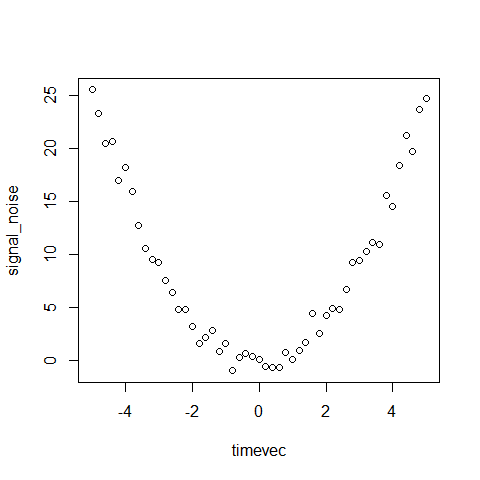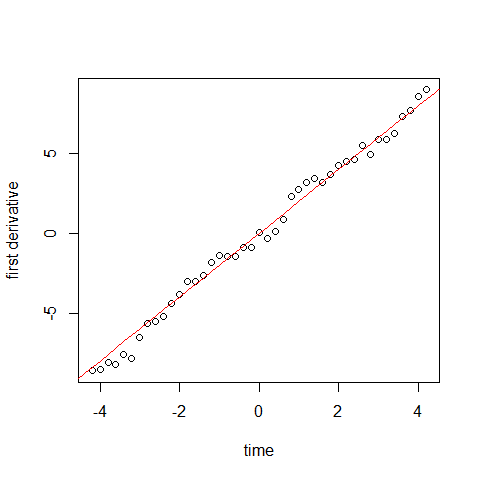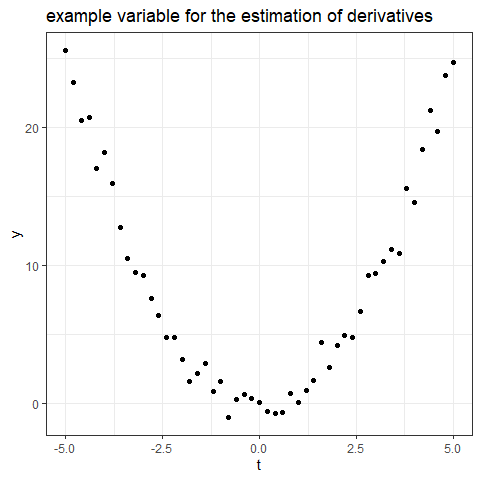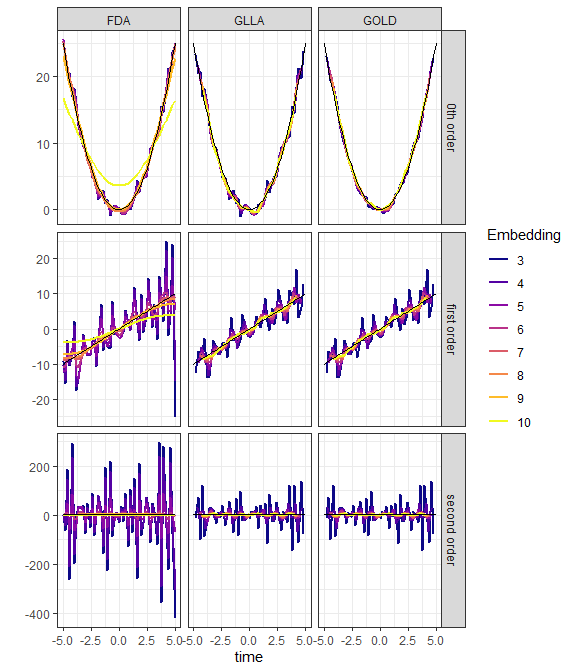library(doremi)

ESTIMATION OF THE DERIVATIVES

There are several way to estimate the derivative of a variable. Three are implemented in the doremi package, with the following functions:

• calculate.glla:, calculates the derivative using GLLA - Generalized Local Linear Approximation - method described . This method allows to estimate the derivatives over a number of measurement points called the embedding number assuming an equally spaced time series.

• calculate.gold:, calculates the derivative using GOLD - Generalized Orthogonal Local Derivative - method described in . The code available on this paper was extracted and adapted for non constant time steps. This method allows calculating over a number of measurement points (called the embedding number) the first and second derivatives (or higher, depending on the order set as input parameter) with errors uncorrelated with the variable.

• calculate.fda:, calculates the derivative using FDA - Functional Data Analysis - method. It generates a B-spline function controlled by a smoothing parameter that fits the outcome to be studied, to then estimates the derivative of that function.

Let’s present a simple example of the use and interest of these functions. Consider a variable of the time following $y(t) = t^2$ with some measurement noise:

timevec <- seq(-5,5,0.2)
noisevec <- rnorm(length(timevec),0,sd = 1)
signal <- timevec^2
signal_noise <- signal + noisevec

plot(timevec,signal_noise)We now that the derivative of this function is $$\dot{y}(t)=2t$$. As an example, we can use the calculate.glla function to estimate the first derivative over 9 points:

der_est <- calculate.gold(time = timevec,
signal = signal_noise,
embedding = 9,
n = 2)

plot(der_est$dtime, der_est$dsignal[,2]
,xlab = "time",
ylab = "first derivative")
lines(timevec,2*timevec,col = 2)Our three functions calculate.gold, calculate.glla and calculate.fda work in a similar manner: We need to give them the time vector, the signal we wish to derivate as a function of the time, the embedding number, and the maximal derivative order n we wish to calculate.

The functions output a dsignal data.frame with n+1 column, each column corresponding to a derivative order (the first column is the zeroth order derivative estimate, the second one the first order derivative etc), and a dtime vector with the corresponding time.

Let’s now construct an example where we can see the effect of the number of point considered to evaluate different derivative order. We construct a long table, were the preceding example is replicated for embedding dimension between 3 and 10, derivative order between 0 and 2, and for the three calculation methods:

conditions <- data.table::CJ(embedding = 3:10,
method = c("GLLA","GOLD","FDA"),
der = 0:2)
derivative_example <- conditions[,.(time = timevec),by = .(embedding,method,der)]
derivative_example[,signal := time^2]
derivative_example[,signal_noise := signal + noisevec]

ggplot2::ggplot(derivative_example)+
ggplot2::geom_point(ggplot2::aes(time,signal_noise))+
ggplot2::theme_bw()+
ggplot2::labs(y = "y",
x = "t",
title = "example variable for the estimation of derivatives")We create a variable representing the true derivative.

# assigning the true derivative for comparison
derivative_example[der  == 1,truder := 2*time]
derivative_example[der  == 2,truder := 2]
derivative_example[der  == 0,truder := signal]

Lets us apply our three functions to estimate the zeroth, first and second order derivative (the zeroth order derivative is a smooth estimate of the variable) for various embedding number and compare with the true derivatives. In our example, we calculate the derivative for each value of embedding. For example, for the gold method, we will do:

derivative_example[method == "GOLD",
derivate := calculate.gold(time = time,
signal = signal_noise,
embedding = embedding,
n = 2)$dsignal[,(der+1)], by = .(embedding,der)] and we will set the time for the derivative in a new variable/ # set the corresponding derivative time derivative_example[method == "GOLD", timeder := calculate.gold(time = time, signal = signal_noise, embedding = embedding,n = 2)$dtime,
by =  .(embedding,der)]

Doing the same for the other methods

# calculation of the derivatives with calculate.glla
derivative_example[method == "GLLA",
derivate := calculate.glla(time = time,
signal = signal_noise,
embedding = embedding,
n = 2)$dsignal[,(der+1)], by = .(embedding,der)] derivative_example[method == "GLLA", timeder := calculate.glla(time = time, signal = signal_noise, embedding = embedding, n = 2)$dtime,
by =  .(embedding,der)]
# calculation of the derivatives with calculate.fda
derivative_example[,spar :=( embedding-3 )/7]
derivative_example[method == "FDA",
derivate := calculate.fda(time = time,
signal = signal_noise,
spar = spar)$dsignal[,(der+1)], by = .(spar,der)] derivative_example[method == "FDA", timeder := calculate.fda(time = time, signal = signal_noise, spar = spar)$dtime,
by = .(spar,der)]

derivative_example[,derlegend := factor(der,levels = 0:2,labels = c("0th order","first order","second order"))]
# plot of the results
ggplot2::ggplot(derivative_example)+
ggplot2::geom_line(ggplot2::aes(timeder,derivate,color = as.factor(embedding)),size = 0.8)+
ggplot2::geom_line(ggplot2::aes(time,truder),color = "black",size = 0.1)+
ggplot2::facet_grid(derlegend~method,scales = "free")+
ggplot2::labs(color = "Embedding",
x = "time",
y = "")+
ggplot2::scale_color_viridis_d(option = "C")+
ggplot2::theme_bw()
#> Warning: Removed 44 row(s) containing missing values (geom_path).In the above example, the derivatives estimated for different embedding number or smoothing parameters are represented in color, and the true derivatives in black. We see that these different functions manage provide an estimation of the derivatives with a precision depending on the embedding number or the smoothing parameter. As visible in the example above with FDA, there is an optimum value of the smoothing parameter or embedding number for which the derivative estimated is the closest to the true derivative.